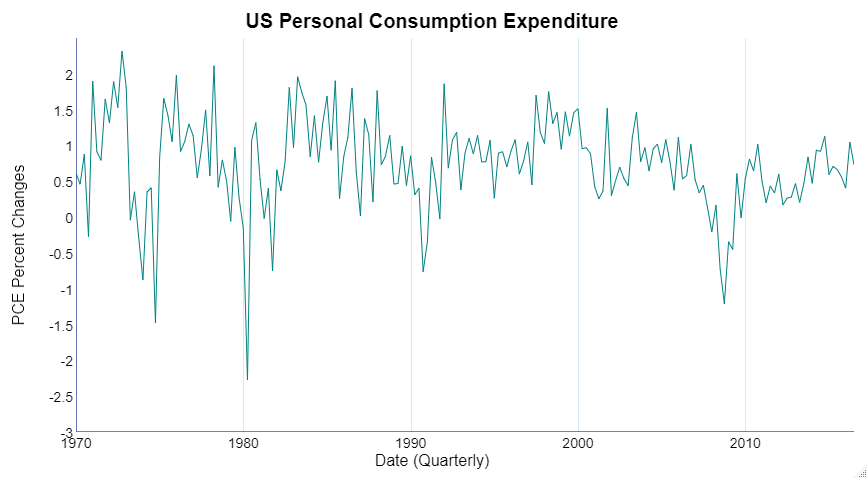# The Bayesians are Coming! The Bayesians are Coming, to Time Series

Original article was published by ODSC – Open Data Science on Artificial Intelligence on Medium# The Bayesians are Coming! The Bayesians are Coming, to Time Series

Editor’s note: Aric is a speaker for ODSC West 2020 this October. Check out his talk, “The Bayesians are Coming! The Bayesians are Coming, to Time Series,” there!

Forecasting has applications across all industries. From needing to predict future values of sales for a product line, energy usage for a power company, to volatility of a portfolio of assets to hedge against risk, forecasting provides needed answers to decision-makers.

Popular approaches to time series forecasting are exponential smoothing models and combinations/variations on autoregressive and moving average models called ARIMA models. Both of these families of approaches are typically done with frequentist statistical methodology compared to Bayesian statistical methodology. Theoretical arguments aside, practitioners benefit from having knowledge of both frequentist and Bayesian time series modeling approaches. The more techniques a practitioner has, the better chance they have at providing the best solution to the decision-maker using the forecasts, which is the true end goal.

Let’s briefly compare these two branches of statistical time series through an example where we try to forecast the percentage change in quarterly United States personal consumption expenditure (PCE) — essentially household buying habits based on the price changes in consumer goods and services. The chart on the left displays the quarterly US PCE from Q1 1970 through Q3 2016. The last seven observations — 2015 and 2016 — were removed and used as a hold-out sample.

At its heart, time series analysis basically tries to break down a series of data into two primary components — signal and noise. We extract the signal from the data and repeat this signal into the future while using the noise to estimate variation in our signal. Specifically, in time series we rely on the assumption that the observations at a certain point in time depend on previous observations in time. But how much emphasis do you put on more recent observations as compared to ones further in the past?

Exponential smoothing models as well as ARIMA class models try to answer this question. The simple (or single) ESM applies a weighting scheme on observations that decreases exponentially the further back in time we go,

where O is bounded between 0 and 1. The larger the value of O, the more that the most recent observation is emphasized as seen in the chart on the right. The above exponentially decreasing weights simplify to the following equation:

These models essentially optimize themselves to forecast one time period into the future.

The following R code computes the simple ESM (forecast package in R needed):

`mod_esm <- ses(train, initial = "optimal", h = 7)`

The forecasts of a simple ESM are rather boring as they are a horizontal line at the forecast for the next time period as we can see below.

Autoregressive (AR) models also forecast a series of observations based solely on past values. The main difference from ESMs is that AR models allow each lag, or previous observation, to not necessarily decreasing exponentially in impact the further back in time you go. To help mitigate numbers of lags in models, combining AR models with moving average (MA) models to form ARIMA models can reduce the parameterization of a model. After using some automatic model selection techniques for time series, we land on the AR(3) model with no MA terms is the best model for our data:

The following R code computes the AR model with three lags (forecast package in R needed):

`mod_ar <- Arima(train, order = c(3,0,0))`

The forecast by the AR(3) model suggests a slight downward projection for future values of PCE as compared to the flat ESM forecast.

Both of the previous models take a frequentist approach to modeling time series. Time series models inherently depend on previous knowledge using lagged variables. The Bayesian framework of modeling relies on previous assumptions about data, which fits in perfectly with time series. The wonderful part about Bayesian time series modeling is that the structures of the models are mostly identical to frequentist models. The main difference is the assumptions. Instead of just taking the inputs into our model — the previous data and parameters — as fixed values we are either using or estimating, we assume that they are random variables with corresponding distributions that we define.

We are going to fit a Bayesian AR(3) model since the AR(3) structure seemed to fit our data the best according to model selection techniques. In Bayesian times series analysis, we estimated the final forecasts through Markov Chain Monte Carlo (MCMC) techniques. The general idea without getting into too much math is that we want to simulate stepping through a series of probabilistic events connected to one another. If we draw enough samples from this series of events, we will eventually get a distribution that resembles the distribution of forecasted future values. For each forecasted future value we actually have a distribution of possible future values at that time point! We take the mean of these distributions at each of the future time points to build our forecast.

The following R code computes the AR model with three lags (bsts package in R needed):

`ss <- AddAutoAr(list(), train, lags = 3, sdy = 1.5)mod_bar <- bsts(train, state.specification = ss, niter = 20000)`

The Bayesian AR(3) model seems to have the closest fit yet of the forecasted models for consumption!

Each of the models had varying forecasts for the future seven quarters of US PCE. Of course, if you want some more details behind the scenes as well as find out which model ending up performing the best, please come see me speak at ODSC West online to learn more about Bayesian time series in data science.